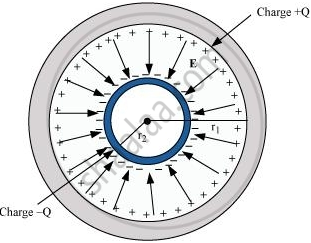Share

# A Spherical Capacitor Consists of Two Concentric Spherical Conductors, Held in Position by Suitable Insulating Supports - CBSE (Science) Class 12 - Physics

#### Question

A spherical capacitor consists of two concentric spherical conductors, held in position by suitable insulating supports (Fig. 2.36). Showwhat the capacitance of a spherical capacitor is given by

C=(4piin_0r_1r_2)/(r_1-r_2)

where r1 and r2 are the radii of outer and inner spheres, respectively.

#### Solution

Radius of the outer shell = r1

Radius of the inner shell = r2

The inner surface of the outer shell has charge +Q.

The outer surface of the inner shell has induced charge −Q.

Potential difference between the two shells is given by,

V=Q/(4piin_0)[1/r_2-1/r_1]

Where,

in_0 = Permittivity of free space

V=Q/(4piin_0)[1/r_2-1/r_1]

=Q(r_1-r_2)/(4piin_0r_2r_1]

Capacitance of the given system is given by

C="Charge(Q)"/"Potenstial difference(V)"

=(4piin_0r_2r_1)/(r_1-r_2)

Hence, proved.

Is there an error in this question or solution?

#### APPEARS IN

NCERT Solution for Physics Textbook for Class 12 (2018 to Current)
Chapter 2: Electrostatic Potential and Capacitance
Q: 29 | Page no. 90

#### Video TutorialsVIEW ALL 

Solution A Spherical Capacitor Consists of Two Concentric Spherical Conductors, Held in Position by Suitable Insulating Supports Concept: Capacitors and Capacitance.
S